# Scaling angles and polygons

Alignments to Content Standards: 7.G.A.1 7.RP.A.2 8.G.A

1. For each shape below, draw a scaled copy with a scale factor of 2. Explain why your drawing is accurate.

1.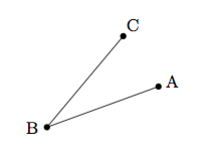2.3.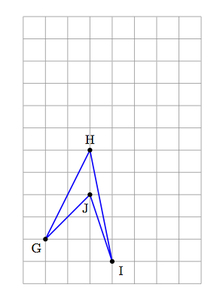2. Below are two pairs of polygons. For each pair, explain whether or not one is a scaled version of the other.

1.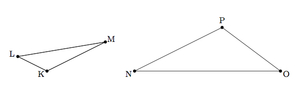2.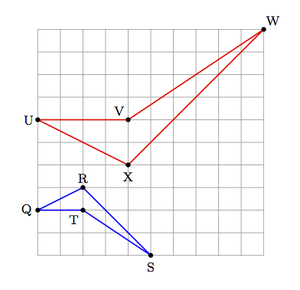## IM Commentary

The goal of this task is to gather together knowledge and skills from the seventh grade in a context which prepares students for the important eighth grade notion of similarity. These important ideas include:

• Scaled shapes have the same angle measures: concretely, when we scale two line segments meeting at a point, the lengths of the segments change but they still are part of the same pair of rays making the same angle.
• Corresponding segments of scaled shapes have proportional lengths. If $a$ and $b$ are side lengths of shape S and $c$ and $d$ are the lengths of the corresponding sides of shape $T$, then $$c:a :: d:b$$ and the common ratio tells us the scale factor going from shape $S$ to shape $T$.

These ideas are sufficient to identify similar polygons and also to produce a scaled version of a given polygon.

The teacher will want to make sure that students have access to rulers, protractors, and possibly patty paper as well to help with their reasoning. In many of the answers, the original angle or polygon is used as part of the scaled version. This is a good technique which saves some time but students may be more comfortable or more inclined to produce a separate scaled angle or polygon. The teacher will need to make sure that students understand that scaling only changes size, not shape: in particular, it does not impact angles. If students try to double the measure of the angle on the first part of (a), the teacher can ask them if the two images are the same shape. The teacher may also encourage students to measure the angles of the shapes in part (a) to verify that they are the same.

Scaled Polygons are one of the most important examples of similar shapes, introduced in 8.G.A. The ideas developed in 7.G.1 and 7.RP.2 are not sufficient, however, to motivate the abstract notion of similarity transformation introduced in the eighth grade. A more abstract, challenging example involving scaled shapes is found in https://www.illustrativemathematics.org/tasks/1818. This task has been aligned with 8.G.A because, while the ideas needed to do this work are available in the seventh grade, the motivation for this example and the synthesis of these important ideas is also appropriate for the eighth grade.

## Solution

1.

1. We can double the length of the two segments as pictured below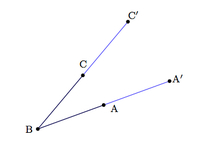(We can physically do this using a ruler, or overlaying some grid paper, or drawing a circle of radius $\overline{CB}$ centered at $C$, etc.)  Regardless of the construction, we then have that segment $\overline{BC'}$ is a scaled version of $\overline{BC}$ with a scale factor of 2 and, similarly, $\overline{BA'}$ is the scaled version of $\overline{BA}$ with scale factor two. Scaling does not change angles and we can see this in the picture as $\angle A'BC'$ is the same as $\angle ABC$.

2. Scaling $\triangle DEF$ can be accomplished as in (i), thinking of $D$, $E$, and $F$ as three points lying on an angle and then drawing in the remaining side after scaling the angle: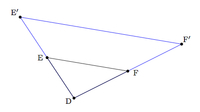We can check by measuring that the length of $\overline{E'F'}$ is twice the length $\overline{EF}$ so all three sides of $\triangle DEF$ have been scaled by a factor of 2.

3. The grid can help draw our scaled quadrilateral as it allows us to measure each side length. Below is a picture of the quadrilateral along with a scaled copy:Starting at vertex G, we make the blue quadrilateral by moving right two units and up 4 units to H, then we move right one unit and down 5 units to I, then moving left one unit and up 3 units to J, finally left two units and down 2 units back to G. If we double all of these numbers, this makes the red quadrilateral starting at its bottom left vertex G$^\prime$. So the red quadrilateral is a scaled version of the blue quadrilateral with scale factor 2.

1. Triangle $NOP$ is not a scaled version of triangle $KLM$. To see why, note that the shortest side of $\triangle KLM$, $\overline{KL}$, is about half the length (perhaps a little less) of the shortest side of $\triangle NOP$, $\overline{PO}$. But the longest side of $\triangle KLM$, $\overline{LM}$, is more than half the length of the longest side of $\triangle NOP$, $\overline{NO}$. This means that there is no possible common scale factor for the sides of these triangles.

Alternatively, we could estimate the angle measures with a protractor and find that they are different for the two triangles. Because of what we found in part (i) of (a), this means that the two triangles are not scaled versions of one another.

2. Starting with vertex Q of the blue quadrilateral we move right two units and up one to vertex R, then right three units and down three units to vertex S, then left three units and up two units to vertex T, and finally left two units back to vertex Q. Starting with vertex U of the red quadrilateral and we go right four units and down two to vertex X, then right six units and up six units to vertex W, then left six units and down four units to vertex V, and finally left four units back to vertex U. If we compare these directions, they are the same except that all lengths have been doubled and up has been replaced with down. So the red shape is a scaled version of the blue shape with a scale factor of two.